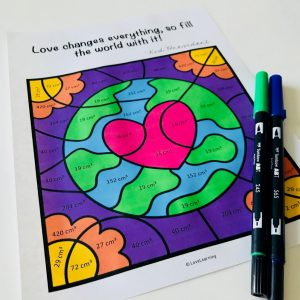# 5 Tips for Teaching Volume

I have always enjoyed teaching volume to my students. It’s one of those interesting math concepts that requires students to use their visual spatial reasoning. I find that a lot of students who struggle with other math concepts like fractions really excel in volume. I love any math concept that can boost a student’s math confidence!

There are a couple of things that I have learned work best for my students when it comes to calculating the volume of prisms and composite figures:

1. Teach all of the different ways to find volume and let students choose the one that works best for them.

There are plenty of different strategies that students can use to calculate volume besides length x width x height.

For example, if the cubes are visible:

1. Count each cube.
2. Count the layers and add them together.
3. Multiply the length x width x height.
4. Calculate the area of the base and multiply it by the height.
Most of my students eventually gravitate towards the formulas, but many also check their work with one of the other strategies.

If not all of the cubes are visible:

1. Count each cube.
2. Find the Length x Width and then add the remaining cubes.
When I ask students to tell me how many more cubes will be needed to fill the prism, some students choose to count all of the empty spaces, and others find the volume of the entire prism and then subtract the visible cubes.

2. Hands-on practice is a must!

When I first start this unit, I teach my students the difference between
1-dimensional, 2-dimensional, and 3-dimensional figures. This truly helps them understand the difference between “squared” and “cubed.”

This year, I also saved empty boxes from my pantry instead of throwing them away and used them for our volume unit. I created several different center activities that my students rotated through, and it was a hit! Giving them the opportunity to explore helped them gain a deep understanding of volume.

For example, in one of the centers, students used Unifix Cubes to fill different small boxes. Here they counted how many cubes were required to fill the box, and then they took the Unifix Cubes out and used the formula “length x width x height” to learn that counting and using the formula result in the same answer.

In another center, I gave students a certain length, width, and height, and they built the prisms and counted the cubes to determine the volume. This really helped engrain which side was the length, and which side was the width (since I often get a lot of questions about the two).

3. Scaffold instruction when teaching volume of composite figures.

For years I made the mistake of teaching my students additive volume of composite figures by simply introducing the topic and then throwing them in to problems with missing sides.

This concept was always a struggle, and I needed to figure out a better way.

This year, I broke down each step for my students and worked slowly through each problem before moving on to more challenging ones.

As you can see in my interactive notes below, we started off by finding all missing sides FIRST. Too often my students would start multiplying whichever numbers they saw without realizing that sides were missing.

Then we focused on finding the volume of each figure at a time, paying special attention to the length, width, and height.

We did the same with the second figure and then added the volume together for the composite figure. I made scaffolded sheets that got harder and harder, but only after students mastered each step. This activity WORKED SO WELL!

What was the biggest mistake that my students made? Multiplication and addition errors. *sigh*

Since this was such an important skill that I wanted my students to master, they had to show me their answers before moving on to the next math activity. If they got anything wrong, I sent them back to revise. They know my policy – we need to learn from our mistakes in math!

4. Allow students to work in partners

One of my favorite ways to use task cards in the classroom are to print them out and tape them around the room. This gets my students moving and I have found that they are much more focused. The purpose of task cards (in my opinion) should be continued practice of a skill through a variety of different questions. I always allow my students to work in partners when completing task cards.

Since students are moving around the room instead of staying in one place, I have a chance to listen to some of their conversations as they get closer to my small group table. Side note – I strategically tape the more challenging problems close to my small group table so that I can hear that discussion. : )

I hear the BEST conversations when my students are working with a partner when their answers don’t match. One partner explains their point of view, and the other naturally explains theirs. This usually results in three scenarios:

1. One of the students changes his/her answer.
2. They both rework the problem to see if their new answer matches.
3. They get frustrated and ask me or another group (if I’m busy) for help.

This right here has SO MUCH value!

5. Have some fun!

Additive volume is pretty labor intensive and hard on the brain. We took a few breaks during this unit and completed:A Color by Number Activity  (which I love because it is self-checking)

I hope these ideas make teaching volume a little easier to understand!

If you are interested in the resources found in this post, see below!

Happy Teaching!